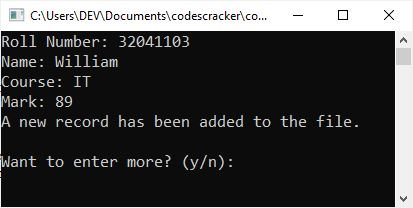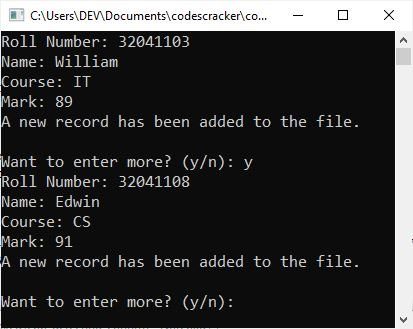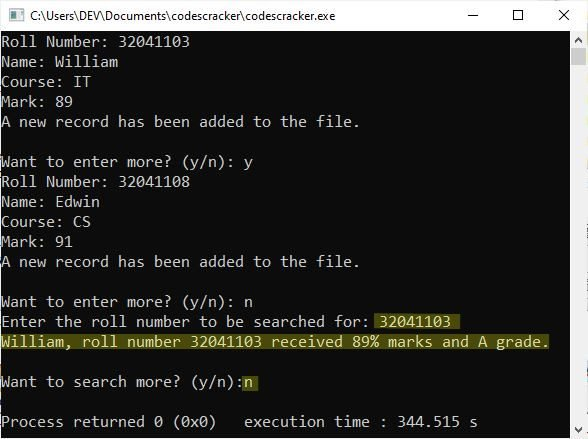# C++ eof() | End-of-File in C++

This post was written and published in order to explain the "eof()" method in C++ using an example program. So, without further ado, let us begin with a brief description.

You can detect when the file has reached its end by using the member function eof() of the "ios" class, which has the prototype:

`int eof();`

It returns non-zero when the end of the file has been reached; otherwise, it returns zero. For example, consider the following code fragment:

```ifstream fin;
fin.open("master.dat", ios::in | ios::binary);

/* As long as eof() is zero,
* that is, the file's end
* is not reached,
* process the file
*/
while(!find.eof())
{
:
}
if(fin.eof())          // if non-zero
cout << "End of file reached!\n";```

The above code fragment processes a file as long as its end-of-file (EOF) is not reached. It uses the eof() function with the stream object to check for the file's end.

To detect the end of a file without using EOF(), you may check whether the stream object has become NULL or not. For example,

```ifstream fin;
fin.open("master.dat", ios::in | ios::binary);
while(fin)
{
:
}```

## C++ EOF Example

Here is an example program demonstrating how to detect the end of a file (EOF) in a C++ program:

```#include <iostream>
#include <fstream>
using namespace std;

class student
{
int rollno;
char name;
char branch;
float marks;

public:
void getdata()
{
cout << "Roll Number: ";
cin >> rollno;
cout << "Name: ";
cin >> name;
cout << "Course: ";
cin >> branch;
cout << "Mark: ";
cin >> marks;

if (marks >= 75)
else if (marks >= 60)
else if (marks >= 50)
else if (marks >= 40)
else
}

void putdata()
{
cout << name << ", roll number " << rollno << " received " << marks << "% marks and ";
}

int getrno()
{
return rollno;
}
} stud1;

int main()
{
ofstream fout("marks.dat", ios::out);

char ans = 'y';
while (ans == 'y' || ans == 'Y')
{
stud1.getdata();
fout.write((char *)&stud1, sizeof(stud1));
cout << "A new record has been added to the file.\n";
cout << "\nWant to enter more? (y/n): ";
cin >> ans;
}
fout.close();

int rno;
char found;
ifstream fin("marks.dat", ios::in);
if (!fin)
{
cout << "Error opening the file!\n";
return 0;
}

ans = 'y';
while (ans == 'y' || ans == 'Y')
{
found = 'n';
cout << "Enter the roll number to be searched for: ";
cin >> rno;

while (!fin.eof())               // end-of-file used here
{
if (stud1.getrno() == rno)
{
stud1.putdata();
found = 'y';
break;
}
}
if (found == 'n')
{
cout << "\nThe entered roll number does not exist in the file.\n";
return 0;
}
cout << "\nWant to search more? (y/n):";
cin >> ans;
}
fin.close();

return 0;
}```

The following snapshot shows the initial output produced by the above program:Now enter the data; for example, type "32041103" as the roll number and press the ENTER key, then "William" as the name and press the ENTER key again, "IT" as the course and press the ENTER key again, and "89" as the mark and press the ENTER key to save these records of a student in the file. The sample output after using these inputs is shown in the following snapshot:Now you can type "y" or "y" to enter the details for another student. For example, see the following snapshot:Now let me type "n" and hit the ENTER key to stop storing the data in the file. Now let me type the roll number and hit the ENTER key to search the student details regarding the given roll number. See the following snapshot:I simply highlighted the text on the output console to draw your attention to the action reflected in the preceding run, ignoring the previous outputs.

#### More Examples

Here are some more examples listed, that uses files in C++ program, you can go for:

C++ Quiz

« Previous Tutorial Next Tutorial »